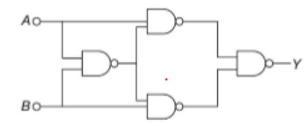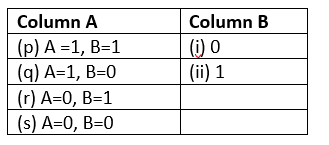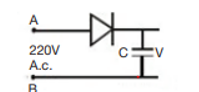Q.1
##### With an increase in temperature, the electrical conductivity of intrinsic semiconductor
• remains unchanged
• increases
• decrease
• first (b) then (c)
Q.2
##### In a p-n junction diode not connected to any circuit
• The potential is the same everywhere
• The p-type side is ay a higher potential than the n-type side
• There is an electric field at the junction directed from the n-type side to the p-type side
• There is an electric field at the junction directed from the p-type side to the n-type side
Q.3
##### In a common base amplifier, the phase difference between the input signal voltage and the output voltage is
• $\frac {\pi}{4}$
• $\pi$
• 0
• $\frac {\pi}{2}$
Q.4
• NOT gate
• NAND gate
• OR Gate
• XOR Gate
Q.5
##### We have a circuit of four NAND gates as shown below Match the column• p -> i, q -> ii ,r->ii, s -> i
• p -> i, q -> ii ,r->ii, s -> i
• p -> i, q -> ii ,r->i, s -> i
• p -> ii, q -> ii ,r->ii, s -> i
Q.6
##### A semiconductor has an electron concentration of $8 \times 10^{13}$ per cm3 and a hole concentration of $5 \times 10^{12}$ per cm3 . The electron mobility is 25000 cm2 V-1 s-1 and the hole mobility is 100 cm2 V-1 s-Then,
• the semiconductor is n-type
• the conductivity is 320 m mho cm-1
• Both (a) and (b)
• None of the above
Q.7
• 12W
• 6 W
• .6 W
• 1,2 W
Q.8
##### In the middle of the depletion layer of the reverse-biased p-n junction, the
• The electric field is zero
• Potential is maximum
• The electric field is maximum
• Potential is zero
Q.9
• 5/8
• 5/4
• 4/5
• 4/7
Q.10
• AND gate
• NAND gate
• NOT gate
• OR gate
Q.11
• a
• b
• c
• d
• e
Q.12
• a
• b
• c
• d
• e
Q.13
• a
• b
• c
• d
• e
Q.14
• a
• b
• c
• d
• e
Q.15
• A
• B
• C
• D
Q.16
##### Which of the following current must be zero in an unbiased PN junction diode?
• Current due to majority charge carriers (both electrons and holes).
• Current due to minority charge carriers (both electrons and holes)
• Current due to majority and minority charge carriers.
• Current due to majority and minority charge carriers (only holes).
Q.17
##### The current  gain of a transistor  in common base arrangement is .The load resistance of the output circuit is $400 k \ohm$ and input resistance is $200 \ohm$, then
• Voltage gain=1900, Power gain=1850
• Voltage gain=1805, Power gain=1900
• Voltage gain=1800, Power gain=1805
• Voltage gain=1900, Power gain=1805
Q.18
##### A 220 V A.C. supply is connected between points A and B. What will be the potential difference V across the capacitor?• 220V
• 110V
• 0V
• $220 \sqrt 2$ V
Q.19
• NOR
• OR
• AND
• XOR
Q.20
##### The breakdown in a reverse-biased p–n junction diode is more likely to occur due to (i) large velocity of the minority charge carriers if the doping concentration is small. (ii) large velocity of the minority charge carriers if the doping concentration is large. (iii) strong electric field in a depletion region if the doping concentration is small. (iv) strong electric field in the depletion region if the doping concentration is large. Which of the below represent the correct options
• (i) and (ii)
• (i) and (iv)
• (iii) and (iv)
• (i) and (iii)